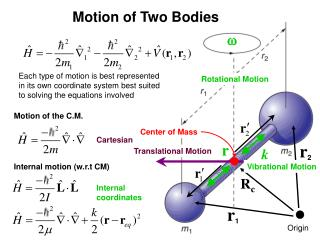Download Presentation18_12afig_PChem.jpg

# 18_12afig_PChem.jpg

Download Presentation## 18_12afig_PChem.jpg

- - - - - - - - - - - - - - - - - - - - - - - - - - - E N D - - - - - - - - - - - - - - - - - - - - - - - - - - -
##### Presentation Transcript

1. 18_12afig_PChem.jpg Motion of Two Bodies w Each type of motion is best represented in its own coordinate system best suited to solving the equations involved Rotational Motion Motion of the C.M. Center of Mass Cartesian r r2 k Translational Motion Internal motion (w.r.t CM) Vibrational Motion Rc Internal coordinates r1 Origin

2. Motion of Two Bodies Centre of Mass Weighted average of all positions Internal Coordinates: In C.M. Coordinates:

3. Kinetic Energy Terms ? ? ? ? ? ? ? ?

4. Centre of Mass Coordinates

5. Centre of Mass Coordinates

6. Centre of Mass Coordinates Similarly

7. Centre of Mass Coordinates

8. Centre of Mass Coordinates Reduced mass

9. Hamiltonian Separable! C.M. Motion 3-D P.I.B Internal Motion Rotation Vibration

10. Rotational Motion and Angular Momentum We rotational motion to internal coordinates Linear momentum of a rotating Body p(t1) p(t2) Ds f Angular Velocity Parallel to moving body Always perpendicular to r Always changing direction with time???

11. Angular Momentum p v f m r w Perpendicular to R and p L Orientation remains constant with time

12. r R Center of mass Rotational Motion and Angular Momentum As p is always perpendicular to r Moment of inertia

13. r R Center of mass Rotational Motion and Angular Momentum

14. r R Center of mass Rotational Motion and Angular Momentum Classical Kinetic Energy

15. r R Center of mass Rotational Motion and Angular Momentum Sincer and p are perpendicular

16. Momentum Summary Classical QM Linear Momentum Energy Rotational (Angular) Momentum Energy

17. Angular Momentum

18. Angular Momentum

19. Angular Momentum in QM

20. Angular Momentum

21. Angular Momentum

22. Two-Dimensional Rotational Motion Polar Coordinates y r f How to we get: x

23. Two-Dimensional Rotational Motion product rule

24. Two-Dimensional Rotational Motion product rule

25. Two-Dimensional Rotational Motion

26. Two-Dimensional Rotational Motion

27. Two-Dimensional Rigid Rotor Assume ris rigid, ie. it is constant As the system is rotating about the z-axis

28. 18_05fig_PChem.jpg Two-Dimensional Rigid Rotor

29. 18_05fig_PChem.jpg Two-Dimensional Rigid Rotor

30. 18_05fig_PChem.jpg Two-Dimensional Rigid Rotor Periodic m = quantum number

31. 18_05fig_PChem.jpg Two-Dimensional Rigid Rotor

32. Two-Dimensional Rigid Rotor m 18.0 12.5 E 8.0 4.5 2.0 0.5 Only 1 quantum number is require to determine the state of the system.

33. Normalization

34. Normalization

35. Orthogonality m = m’ m ≠ m’ 18_06fig_PChem.jpg

36. 14_01fig_PChem.jpg Spherical Polar Coordinates ?

37. 14_01fig_PChem.jpg Spherical Polar Coordinates

38. 14_01fig_PChem.jpg The Gradient in Spherical Polar Coordinates Gradient in Spherical Polar coordinates expressed in Cartesian Coordinates

39. 14_01fig_PChem.jpg The Gradient in Spherical Polar Coordinates Gradient in Cartesian coordinates expressed in Spherical Polar Coordinates

40. 14_01fig_PChem.jpg The Gradient in Spherical Polar Coordinates

41. 14_01fig_PChem.jpg The Gradient in Spherical Polar Coordinates

42. 14_01fig_PChem.jpg The Laplacian in Spherical Polar Coordinates Radial Term Angular Terms OR OR

43. Three-Dimensional Rigid Rotor Assume ris rigid, ie. it is constant. Then all energy is from rotational motion only.

44. 18_05fig_PChem.jpg Three-Dimensional Rigid Rotor Separable?

45. Three-Dimensional Rigid Rotor k2= separation Constant Two separate independent equations

46. 18_05fig_PChem.jpg Three-Dimensional Rigid Rotor Recall 2D Rigid Rotor

47. 18_05fig_PChem.jpg Three-Dimensional Rigid Rotor This equation can be solving using a series expansion, using a Fourier Series: Legendre polynomials Where

48. Three-Dimensional Rigid Rotor Spherical Harmonics

49. The Spherical Harmonics For l=0, m=0

50. The Spherical Harmonics For l=0, m=0 Everywhere on the surface of the sphere has value what is ro ? r = (ro, q, f)